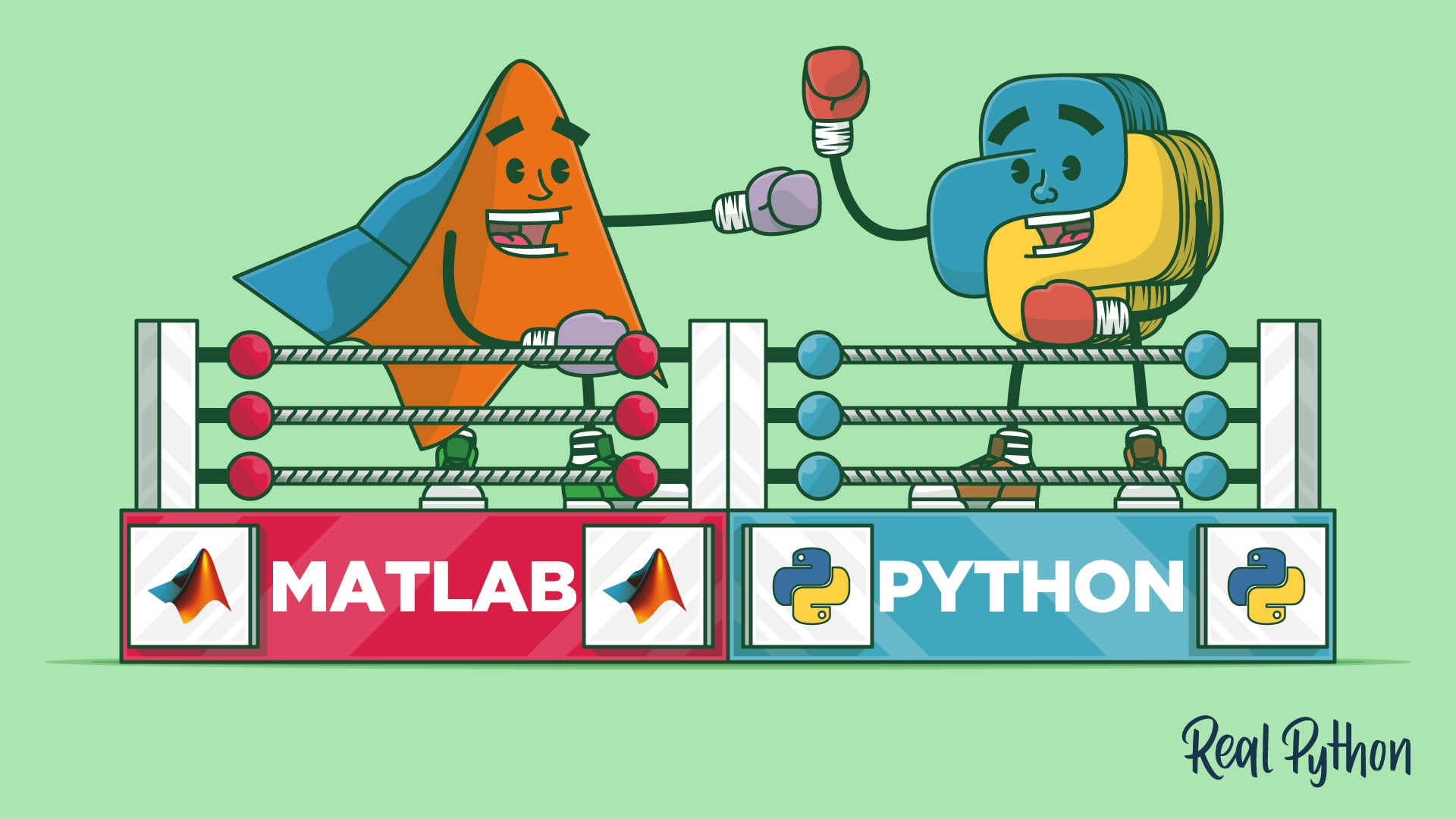# MATLAB vs Python: Why and How to Make the SwitchLearn about MATLAB vs Python, why and how you should switch from MATLAB to Python. Evaluate the differences of using MATLAB vs Python. Convert scripts from MATLAB to Python. MATLAB® is widely known as a high-quality environment for any work that involves arrays, matrices, or linear algebra.

In this step-by-step tutorial, you'll learn about MATLAB vs Python, why you should switch from MATLAB to Python, the packages you'll need to make a smooth transition, and the bumps you'll most likely encounter along the way.

MATLAB® is widely known as a high-quality environment for any work that involves arrays, matrices, or linear algebra. Python is newer to this arena but is becoming increasingly popular for similar tasks. As you’ll see in this article, Python has all of the computational power of MATLAB for science tasks and makes it fast and easy to develop robust applications. However, there are some important differences when comparing MATLAB vs Python that you’ll need to learn about to effectively switch over.

• Evaluate the differences of using MATLAB vs Python
• Set up an environment for Python that duplicates the majority of MATLAB functions
• Convert scripts from MATLAB to Python
• Avoid common issues you might have when switching from MATLAB to Python
• Write code that looks and feels like Python

## Top Python Development Companies | Hire Python Developers

After analyzing clients and market requirements, TopDevelopers has come up with the list of the best Python service providers. These top-rated Python developers are widely appreciated for their professionalism in handling diverse projects. When...

## Python Hello World Program - Create & Run Your First Python Program in PyCharm

Python Hello World Program - Your first step towards Python world. Learn how to create the Hello World Python program in PyCharm.

## Python GUI Programming Projects using Tkinter and Python 3

Python GUI Programming Projects using Tkinter and Python 3

## Guide to Python Programming Language

Guide to Python Programming Language

## Tutorial How to write MatLab functions in Python

A tutorial on writing MatLab-like functions using the Python language and the NumPy library.